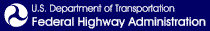Skip to content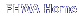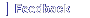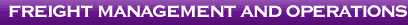# Appendix E: Results of the Vehicle Stability and Control Analysis

This appendix documents the performance metrics used to study vehicle stability and control for five maneuvers that were simulated in TruckSim®. Each run of TruckSim® produced an output data file. These files were analyzed with Matlab to calculate the desired performance parameters. Table 24 in Section 3.2 of the main text lists the performance metrics that were extracted from each of the five maneuvers and the peril that each is intended to assess. Appendix C documents how these performance metrics were calculated.

The following pages are graphs of various performance metrics for each simulated test maneuver. On each page, the test maneuver is indicated on the left, the performance metric is indicated on the top, and additional test parameters such as brake failure is indicated on the right. The eight plots on every page correspond to the eight vehicle configurations under investigation.

The value above the plot is the result of the simulation. It is the number in the corresponding table of results in Section 3.3 of the main text.

The avoidance maneuver required a family of similar paths. Specifically, eight lane change widths (3, 6, 9, 12, 15, 18, 21, and 24 ft.) were simulated. These eight lane changes correspond to eight excitation frequencies, as shown in Table C1 in Appendix C. From these eight excitation frequencies, the highest responses (i.e. peak off-tracking, peak rearward amplification, and peak load transfer ratio) are reported in this appendix and in Table 29 of the main text.

Figure E-1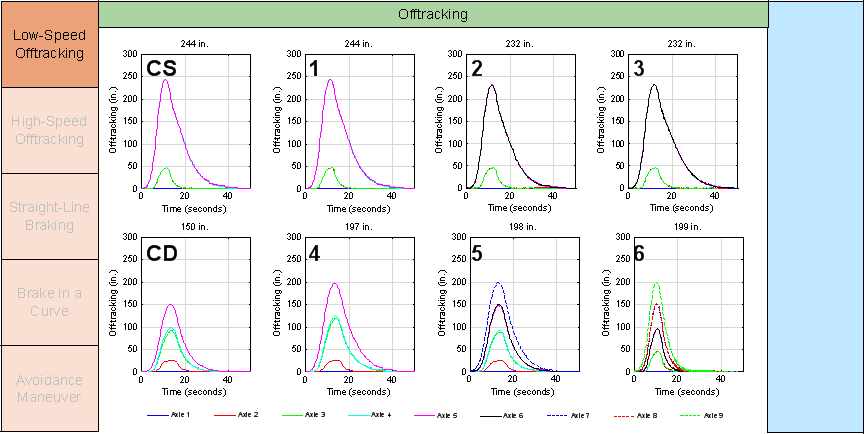Figure E-2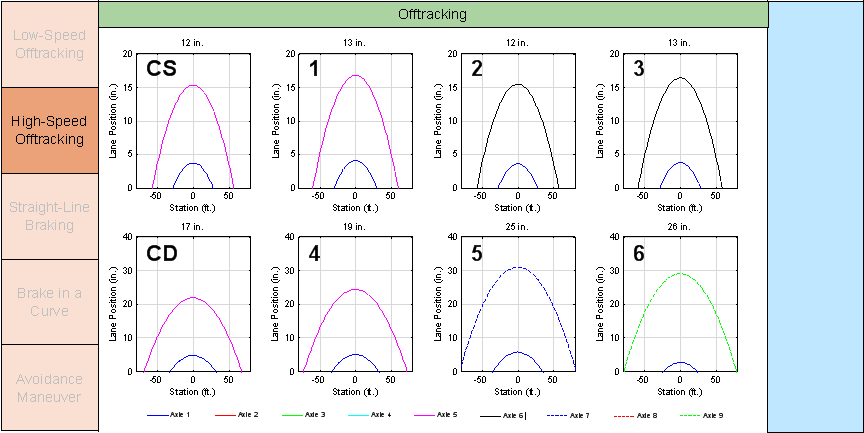Figure E-3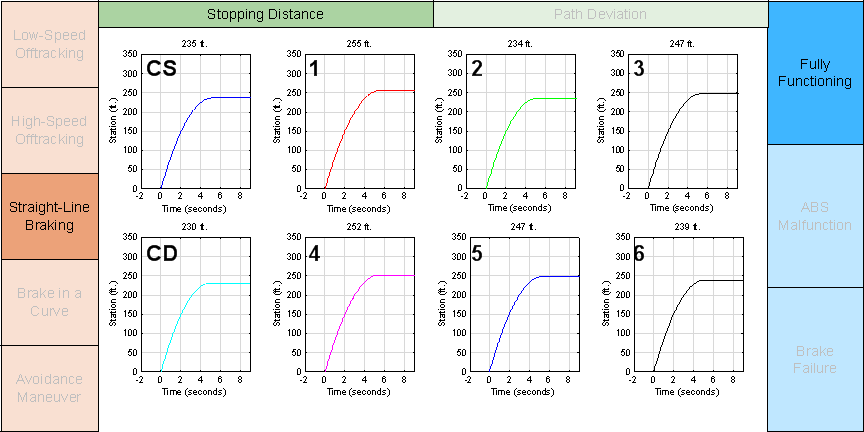Figure E-4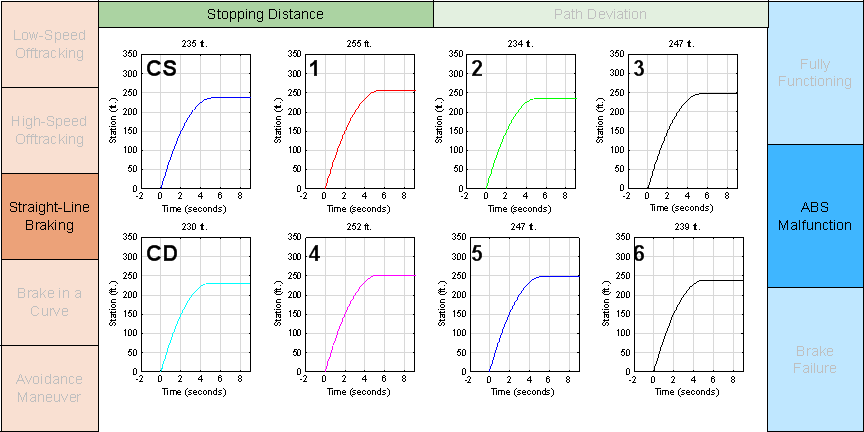Figure E-5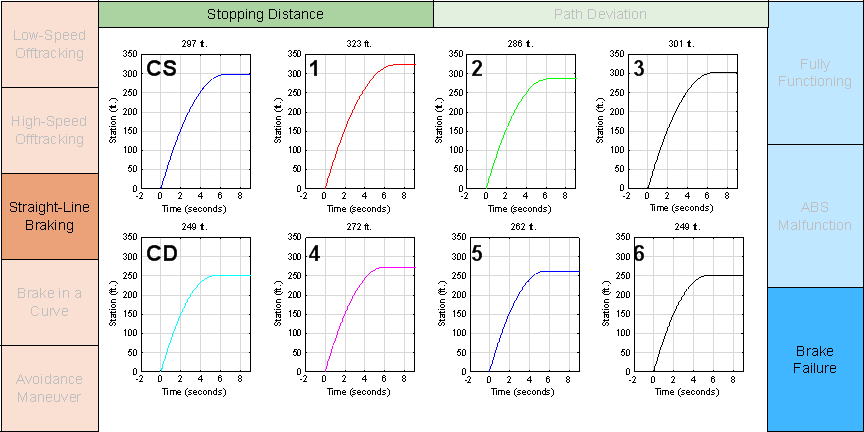Figure E-6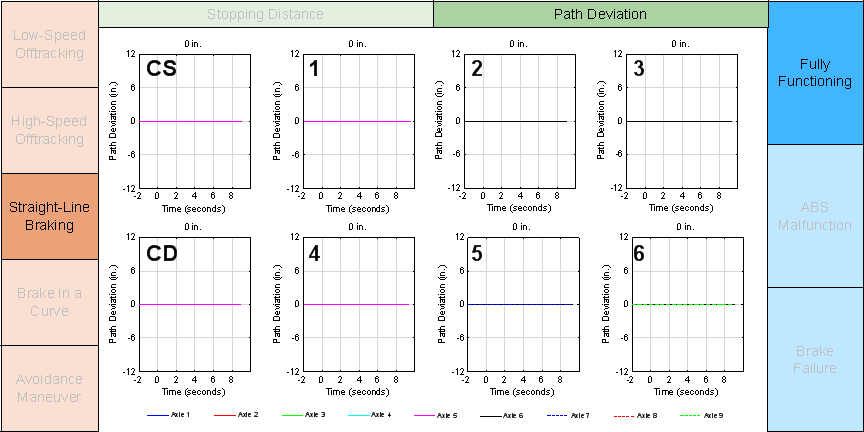Figure E-7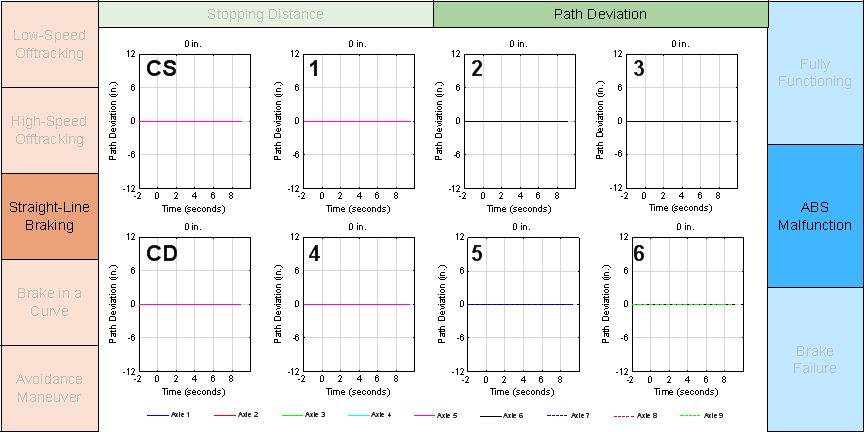Figure E-8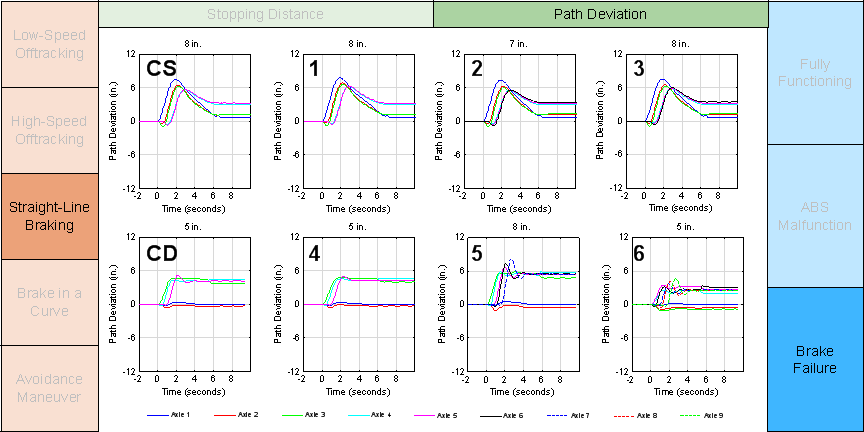Figure E-9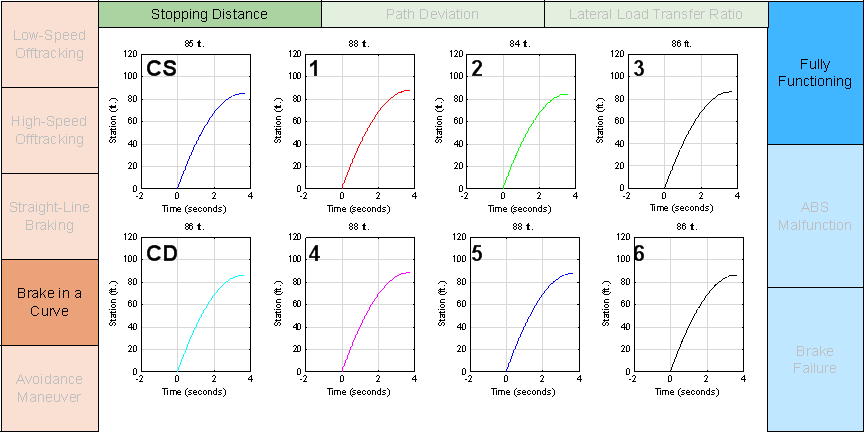Figure E-10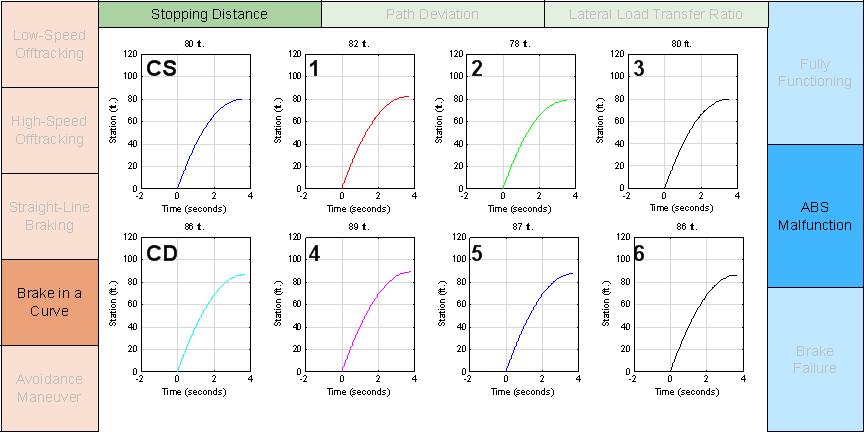Figure E-11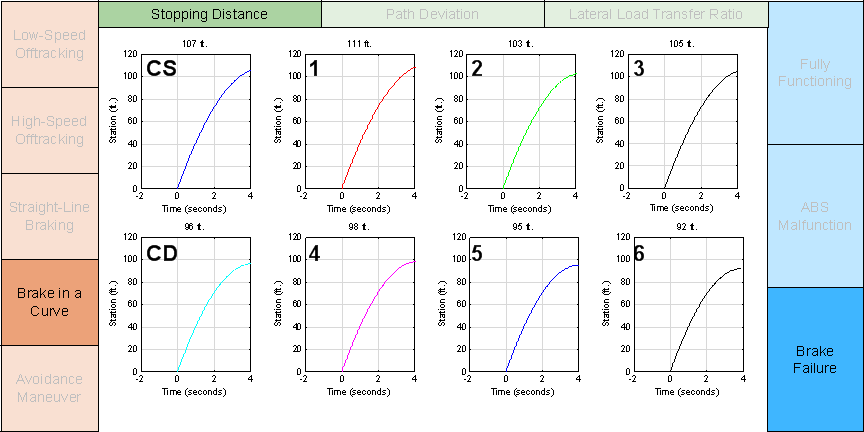Figure E-12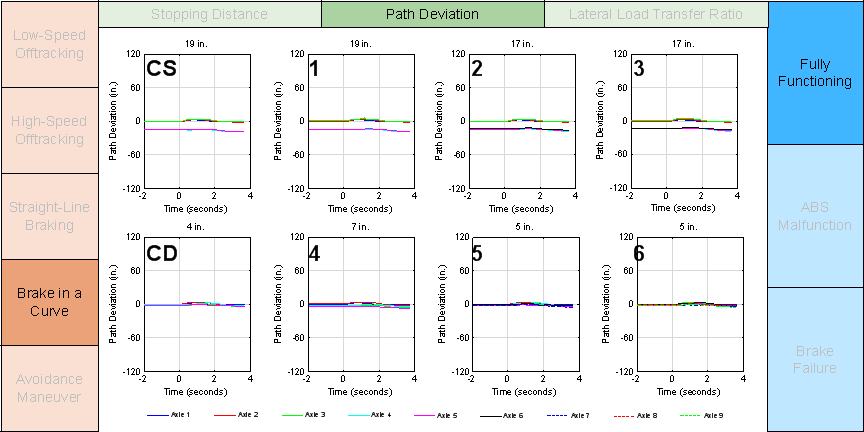Figure E-13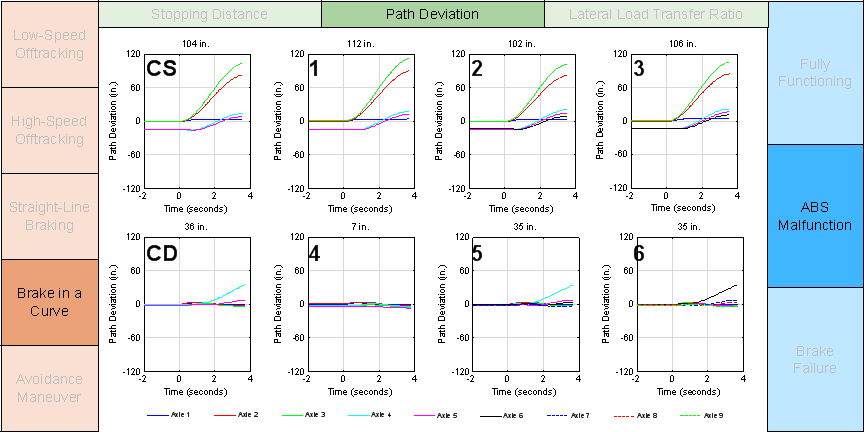Figure E-14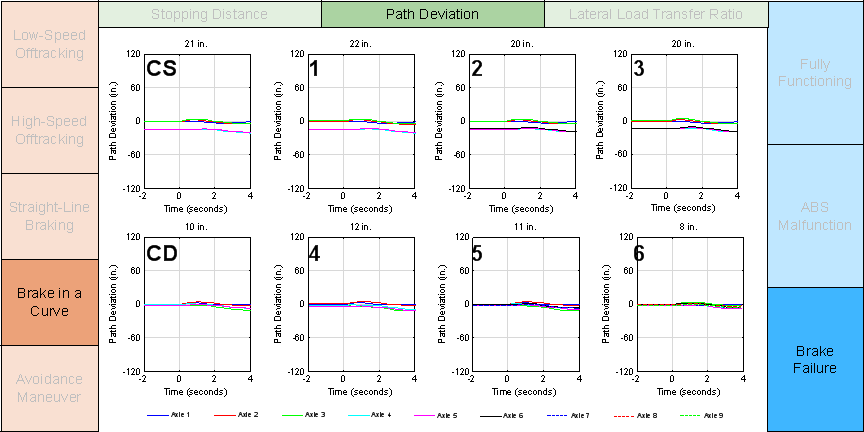Figure E-15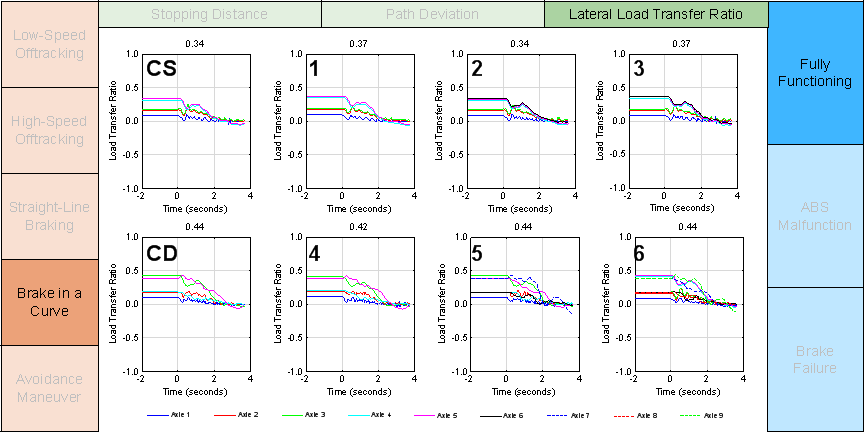Figure E-16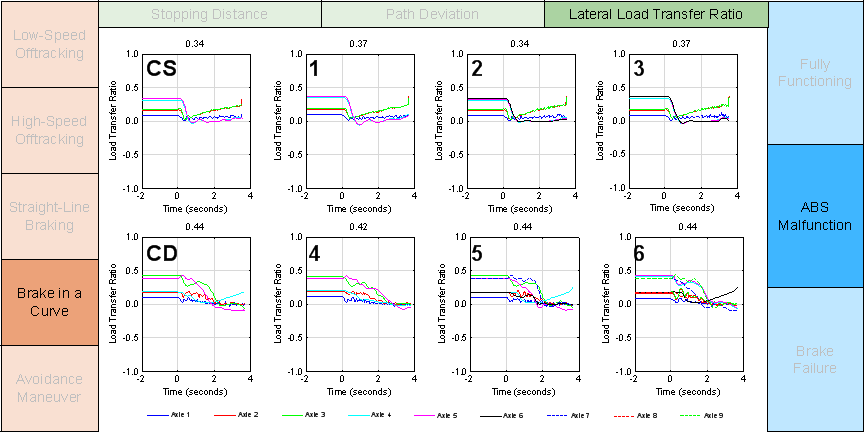Figure E-17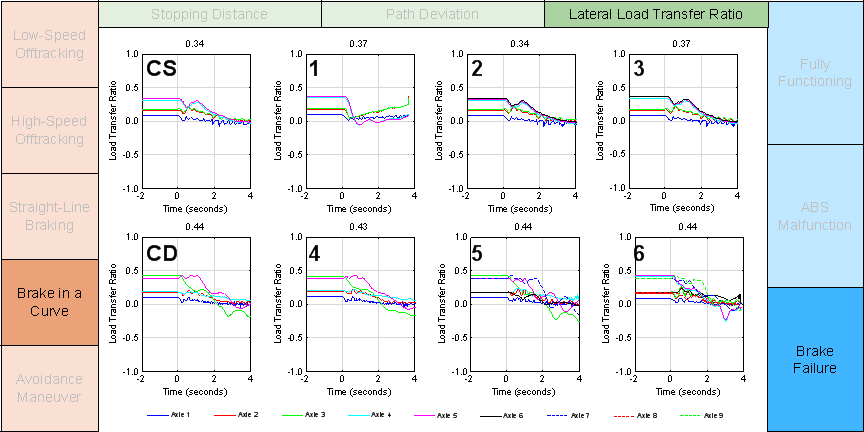Figure E-18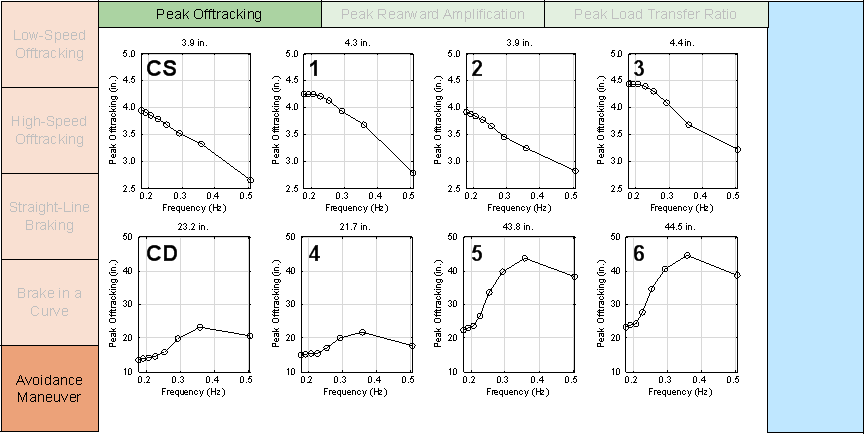Figure E-19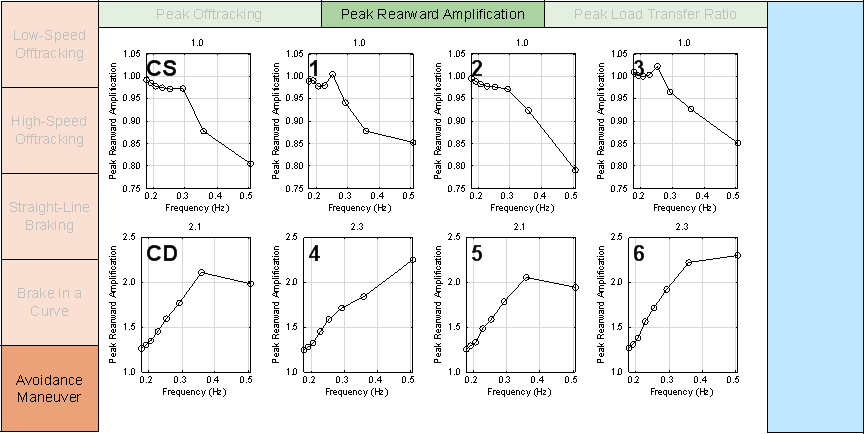Figure E-20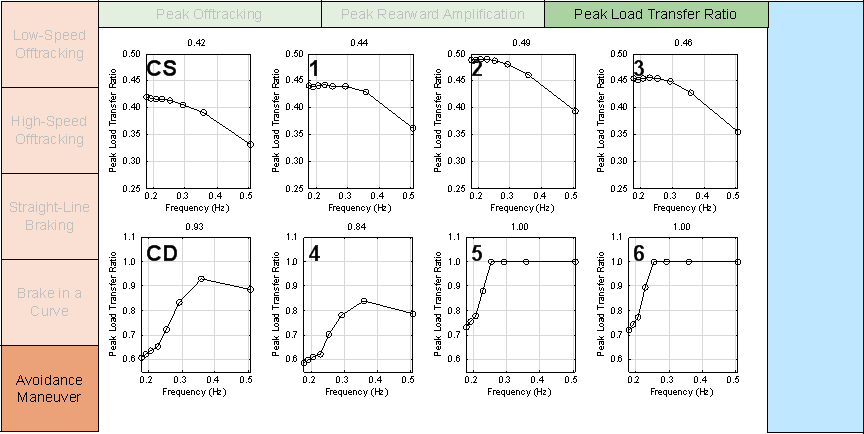previous | next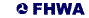United States Department of Transportation - Federal Highway Administration